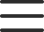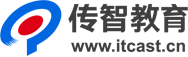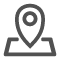# 传智播客java就业班入学测试题

1. 在JAVA中，下列哪些说法是正确的( )

A.java源文件的扩展名为.java

B.写好的java源程序可以直接运行

C.编写的源程序必须先编译后才能运行

D.程序员可以读懂扩展名为.class的文件

2. 下列标识符哪个是合法的( )

A.class

B.\$abc

C.1234

D._name

3. 下面哪些赋值语句是正确的?( )

A. long test = 012;

B. float f = -412;

C. int other = (int) true;

D. double d = 0x12345678;

E. byte b = 128;

4. 在Java中，下列语句不能通过编译的有( )

A. String s= “join”+ 3;

B. int a= “join”+3;

C. int a= ‘a’+5;

D. float f=5+5.5;

5. 设 a = 3，则表达式 (--a )<

A. 16

B. 8

C. 24

D. 12

6. 下列代码运行的结果是( )

public static void main(String[] args) {

int x = 4;

int y = 5;

if(x++>4 & ++y>5) {

x++;

}

System.out.println("x=" + x + ",y=" + y);

}

A. x=4,y=5

B. x=4,y=6

C. x=5,y=6

D. x=6,y=6

7. 尝试运行下面的代码会发生什么?( )

public class Test {

public static void main(String[] args) {

boolean m = true;

if (m = false) {

System.out.println("true");

} else {

System.out.println("false");

}

}

}

A. 输出字符串”true”

B. 输出字符串”false”

C. 编译时错误

D. 运行时错误

8. 编译并运行以下代码将发生什么? ( )

public static void main(String args[]){

char digit = 'a';

for (int i = 0; i < 10; i++){

switch (digit)

{

case 'x' :

{

int j = 0;

System.out.println(j);

}

default :

{

int j = 100;

System.out.println(j);

}

}

}

int i = j;

System.out.println(i);

}

A. 输出11次100

B. 输出10次100，然后抛出运行期异常

C. 编译错误，因为变量i不能在main() 方法中被声明2次

D. 编译错误，因为变量j不能在main() 方法中被声明2次

E. 以上都不对

9. class Demo

{

public static int fun(int c)

{

return c+=2;

}

public static void main(String[] args)

{

int temp = fun(2);

System.out.println(temp);

}

}

A. 2

B. 4

C. 6

D. 8

10. 下面哪些函数是public void aMethod(){...}的重载函数?( )

A. void aMethod( ){...}

B. public int aMethod(int a, float b){...}

C. public void aMethod (){...}

D. public float aMethod (int m){…}

11. 在java中，关于数组描述正确的是( )

A.数组中的索引下标从1开始

B.存储在数组当中的数据都属于同一数据类型

C.通过数组名.length()能获得数组的长度

D.数组的最大索引下标是数组的长度减1

12. 下面程序的输出结果是什么( )

int[] arr = new int;

System.out.println(arr);

A.编译不通过

B.运行时出错

C.输出null

D.输出0

13. 下面哪个语句正确地声明一个整型的二维数组( )

A. int a[][] = new int[][];

B. int b = new int[][];

C. int c[][] = new int;

D. int []d[] = new int;

14. 以下代码输出是( )

class Demo {

public static void main(String[] args) {

int i = 0;

int sum = 0;

while (i <= 10) {

i++;

if (i % 2 != 0)

continue;

sum += i;

}

System.out.println(sum);

}

}

A. 55

B. 45

C. 35

D. 30

15. 和下面代码能完成相同的选项是()

class Demo {

public static void main(String[] args) {

int i = 1;

int sum = 0;

while (i <= 100) {

if (i % 2 == 0) {

sum = sum + i;

}

i++;

}

System.out.println(sum);

}

}

A. for (int x =1; x<=100;x++){ sum=sum+x;}

B. for (int x =0; x<=100;x+=2){ sum=sum+x;}

C. for (int x =1; x<=100;x+=2){ sum=sum+x;}

D.上述全对

16. 下列有关类、对象和实例的叙述，正确的是哪一项?()

A.类就是对象，对象就是类，实例是对象的另一个名称，三者没有差别

B.对象是类的抽象，类是对象的具体化，实例是对象的另一个名称

C.类是对象的抽象，对象是类的具体化，实例是类的另一个名称

D.类是对象的抽象，对象是类的具体化，实例是对象的另一个名称

17. 下面关于java中包的说法正确的是( )

A. 在java中可以使用import语句导入包

B. 在java中可以使用package语句导入包

C. 位于同一个包中的类，不需要导包就可以直接访问

D. 不同的包中可以出现类名相同的类

18. 下列有关抽象类的叙述正确的是哪项?()

A.抽象类中一定含有抽象方法

B.抽象类既能被实例化也能被继承

C.抽象类的声明必须包含abstract关键字

D.抽象类中不能有构造方法

19. 下列有关接口的叙述错误的是哪项?()

A.接口中只能包含抽象方法和常量

B.一个类可以实现多个接口

C.类实现接口时必须实现其中的方法

D.接口不能被继承

20. 运行以下代码片段，输出结果是?( )

class X {

Y b = new Y();

X() {

System.out.print("X");

}

}

class Y {

Y() {

System.out.print("Y");

}

}

public class Z extends X {

Y y = new Y();

Z() {

System.out.print("Z");

}

public static void main(String[] args) {

new Z();

}

}

A. Z

B. YZ

C. XYZ

D. YXYZ

21. 下列关于类的继承的描述，正确的有( )

A. 一个类可以同时继承多个父类

B. 一个类可以具有多个子类

C. 子类会自动拥有父类所有的方法

D. 一个类继承另一个类需要使用 extends 关键字

22. 下列选项中关于java中this关键字的说法错误的有( )

A. this关键字是一个对象的引用

B. this关键字可以用于引用当前类以外其他类型的对象

C. this可用于构造函数中，调用类本身重载的构造函数，但是必须写在首行

D. this可用于静态方法中

23. 下列关于构造函数的说法正确的是( )

A. 方法名必须与类名相同

B. 使用new关键字创建对象时，java虚拟机会自动调用构造函数

C. 我们在定义一个类时，必须要声明至少一个构造函数

D. 构造函数中不能使用return语句

24. 编译并运行以下代码将发生什么?( )

class MyClass {

int x;

MyClass(int i) {

x = i;

}

public static void main(String args[]) {

MyClass m1 = new MyClass(100);

MyClass m2 = new MyClass(100);

if (m1.equals(m2)) {

System.out.println("Both are equal");

} else {

System.out.println("Both are not equal");

}

}

}

A. 代码编译时报出错误提示信息“equals() 方法未定义”

B. 编译通过，抛出运行期异常.

C. 输出Both are equal.

D. 输出Both are not equal

25. 运行以下的main()方法，结果是?( )

1 public static void main(String[] args)

2 {

3 String myString;

4 int x = 100;

5

6 if (x < 100) myString = "x is less than 100";

7 if (x > 100) myString = "x is greater than 100";

8 System.out.println(myString.length());

9 }

A. 编译时报出错误提示信息“变量myString没有被初始化”

B. 编译通过

C. 编译未能通过。但如果变量myString在第8行前的代码中被初始化，代码可以编译通过，运行时可以输出字符串myString的长度

D. 以上都不对

26. 有如下代码，请问哪些是正确的?()

class ClassA{}

class ClassB extends ClassA{}

class ClassC extends ClassA{}

ClassA p0 = new ClassA();

ClassB p1 = new ClassB();

ClassC p2 = new ClassC();

ClassA p3 = new ClassB();

ClassA p4 = new ClassC();

A.p0 = p1;

B.p1 = p2;

C.p1 = (ClassB)p3;

D.p2 = (ClassC)p4;

27. 关于继承, 以下说法正确的是: ()

A.Java中只支持单继承, 一个类只能继承一个类, 但是可以有多个子类

B.一个类如果没有自己写无参构造方法, 那么子类将无法继承

C.子类可以当父类用, 父类不可以当子类用

D. 子类重写父类方法时访问权限不能更低

28. 编译并运行以下代码的输出结果是?( )

String s1 = new String("amit");

System.out.println(s1.replace('m','r'));

System.out.println(s1);

String s3 = "arit";

String s4 = "arit";

String s2 = s1.replace('m','r');

System.out.println(s2 == s3);

System.out.println(s3 == s4);

A. arit

amit

false

true

B. arit

arit

false

true

C. amit

amit

false

true

D. arit

amit

true

true

29. 下列哪些代码可以顺利通过编译和运行? ( )

A.Integer i = new Integer('A');

B.Integer i = new Integer("7");

C.Character c = new Character("A");

D.Boolean b = new Boolean(null);

E.Integer i = new Integer("0x10");

30. 在Java中，如果要在字符串类型对象s="java"中，得到字符 'v' 出现的位置，可使用下面的那个方法?( )

A. s.matches('v');

B. s.charAt('v');

C. s.indexOf('v');

D. s.substring('v');

A. ArrayList集合底层是数组实现，该集合线程不安全

B. Vector 集合元素的存放是无序的

D. 这三个集合都是List接口的实现类

32. 下面程序的输出结果是什么( )

Set set= new HashSet();

System.out.println(set.size());

A. 编译不通过

B. 运行时出错

C. 输出3

D. 输出2

B. removeFirst()

C. remove(Object o)

34. 下列哪些集合属于Collection体系的子类( )

A. TreeMap

B. ArrayList

C. Hashtable

D. HashSet

35. 下面有关Java异常处理的说法错误的是( )

A. 一个try块只能有一条catch语句

B. 一个try块中可以不使用catch语句

C. catch块不能单独使用，必须始终与try块在一起

D. finally块不能单独使用，必须始终与try块在一起

36. 下列关键字能和try语句一起使用的是( )

A. final

B. finalize

C. finally

D. catch

37. 下面程序的运行结果是( )

FileOutputStream fos = new FileOutputStream(“c:\\demo.txt”);

fos.write(“abc”);

fos.close();

A.在C盘创建文件demo.txt,但文件是空的

B.在C盘创建文件demo.txt,并写入数据abc

C.将C盘已有的文件demo.txt中追加写入abc

D.编译失败

38. 下列关于Java中文件输入输出的说法正确的是( )

A. 在Java中，文件的输入输出功能是通过流来实现的

B. 如果要把数据写入到一个文件中，需要创建一个输入流对象

C. 字符流在某些方面比如汉字的处理，比字节流更方便

39. 请看如下代码：

BufferedWriter bw = new BufferedWriter(new FileWriter("d:\\b.txt"));

String line = null;

while ((line = br.readLine()) != null) {

bw.write(line);

bw.newLine();

bw.flush();

}

bw.close();

br.close();

A. 把c盘目录下的a.txt文件内容复制到d盘目录下的b.txt

B. 把d盘目录下的b.txt文件内容复制到c盘目录下的a.txt

C. 读取c盘目录下a.txt文件，输出在控制台

D. 把控制台的内容写入到d盘目录下的b.txt文件中

40. 关于泛型的说法正确的是()

A.泛型是JDK1.5出现的新特性

B.泛型是一种安全机制

C.使用泛型避免了强制类型转换

D.使用泛型必须进行强制类型转换

41. 关于方法的递归算法，说法正确的是()

A.递归就是方法自己调用自己

B.递归的次数不能过大，否则会导致栈内存溢出

C.使用递归算法，方法必须有返回值

D.构造方法不可以使用递归算法

42. 下列哪个关键字可以用于实现同步?( )

A. native

B. static

C. synchronized

D. abstract

43. 下列哪些方法可以让线程中断? ( )

A. sleep

B. start

C. notify

D. wait

44. 启动线程调用哪个方法? ( )

A. run()

B. start()

C. play()

D. go()

45. 在 awt 中，下列哪个组件可以实现带标题的窗口( )

B. Button

C. Frame

D. TextField

46. 编写Button组件的事件处理类时，需要实现哪个接口? ( )

A. ItemListener

B. ActionListener

C. ButtonListener

D. WindowListener

47. 下面哪项不是TCP的特点? ( )

A.面向连接

B.数据不可靠

C.传输速度慢

D.对数据大小无限制

48. TCP协议的服务器的Socket对象是下面哪一个类? ( )

A. Socket

B.InputStream

C.ServerSocket

D.OutputStream

49. 正则表达式中可以表示所有的单词字符信息的是下面哪个规则：( )

A. \W

B. \w

C. [a-zA-Z]

D.[a-zA-Z_0-9]

50. 获取Class类型的对象的三种方式是：( )

A. Object类的getClass()

B. class静态属性

C. 自己创建Class对象

D. Class类的forName()静态方法